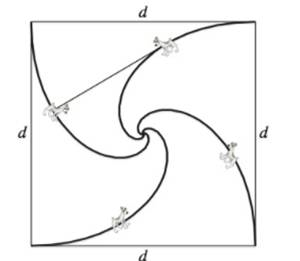Chapter 10, Problem 13PS

Chapter
Section
Textbook Problem

Finding a Polar Equation Four dogs are located at the corners of a square with sides of length d. The dogs all move counterclockwise at the same speed directly toward the next dog, as shown in the figure. Find the polar equation of a dog’'s path as it spirals toward the center of the square.To determine

To calculate: The polar equation that describes the dog’s path.

Explanation

Given: Four dogs located at the corners of the square of length d move towards their neighbor in the anti-clockwise direction as shown:

Formula used:

The slope of the tangent line of the polar curve r=f(θ) at the point (r,θ) is:

m=f(θ)cosθf'(θ)sinθf(θ)sinθ+f'(θ)cosθ

Calculation:

Consider the dog located in the first quadrant.

The coordinates of the dog are (r,θ).

The coordinates of its neighbor to the left are (r,θ+π2).

Convert the polar coordinates into the Cartesian coordinates:

(x1,y1)=(rcosθ,rsinθ)(x2,y2)=(rcos(θ+π2),rsin(θ+π2))=(rsinθ+rcosθ)

The slope of line joining the two points willbe:

m=rcosθrsinθrsinθrcosθ=sinθcosθsinθ+cosθ

This should be equal to the slope of the tangent line at the point (r,θ)

Still sussing out bartleby?

Check out a sample textbook solution.

See a sample solution

The Solution to Your Study Problems

Bartleby provides explanations to thousands of textbook problems written by our experts, many with advanced degrees!

Get Started

Sketch the graphs of the equations in Exercises 512. xy=2x3+1

Finite Mathematics and Applied Calculus (MindTap Course List)

Use the guidelines of this section to sketch the curve. y=1x+lnx

Single Variable Calculus: Early Transcendentals, Volume I

Solve sin2xsinx=0 if 0x2.

Trigonometry (MindTap Course List)

is the binomial series for:

Study Guide for Stewart's Single Variable Calculus: Early Transcendentals, 8th

Multiply 56423 .

Mathematics For Machine Technology